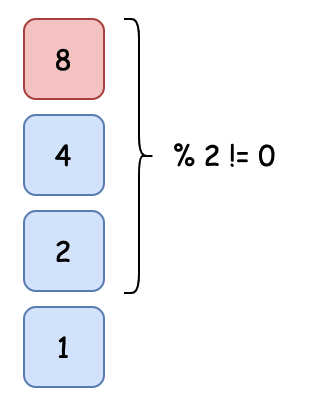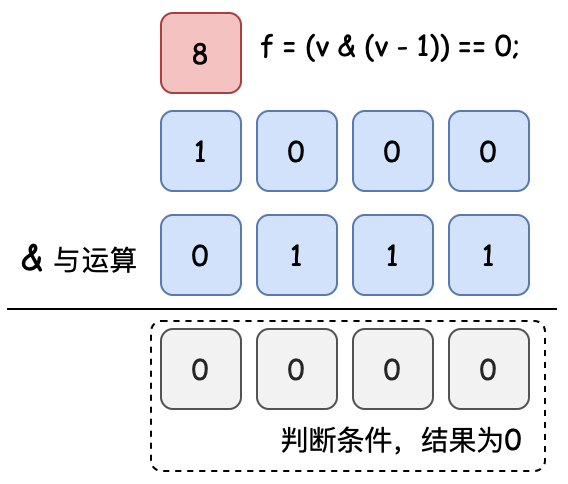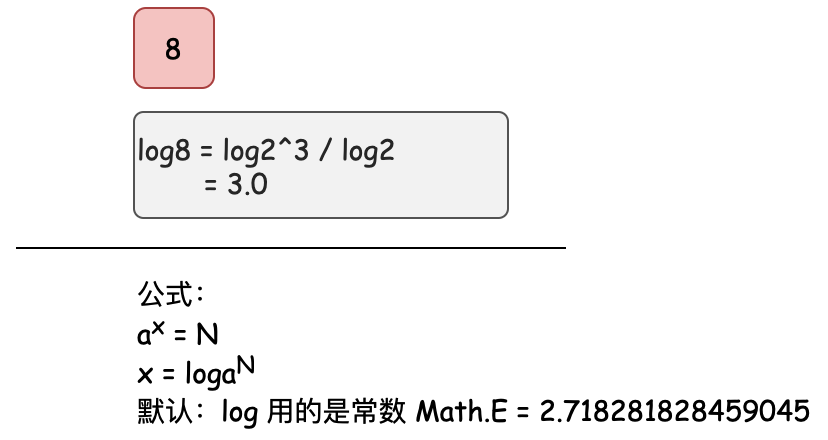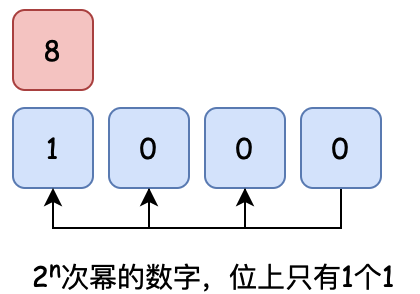# # 《程序员数学：判断2次方数》—— 除法、二进制、对数，你会用哪种方式判断？

## # 一、前言

`每一个知识的索引都可能会牵扯出一片的内容`

## # 二、判断2次方数

🤔那除此之外还有其他手段吗？我们接下来就分别实现下

### # 1. 整除法``````public boolean isPowerOfTwo01(int number) {
if (number < 1) return false;

int dividedNumber = number;
while (dividedNumber != 1) {
if (dividedNumber % 2 != 0) {
return false;
}
dividedNumber = dividedNumber / 2;
}

return true;
}
``````
1
2
3
4
5
6
7
8
9
10
11
12
13
• 循环数字除以2的结果与2求模计算看余数是否为0，只要有一次不为0，那么就不是2的次方数。

### # 2. 二进制``````public boolean isPowerOfTwo02(int number) {
if (number < 1) return false;
return (number & (number - 1)) == 0;
}
``````
1
2
3
4
• 在斯坦福大学的计算资料中，有这么一条关于判断2的次方数的内容；`f = (v & (v - 1)) == 0;` 错位进行 & 与运算，结果为0则是2次方数【过程如图】。
• 此外这里我们过滤了小于的数字，如果不过滤则需要使用；`f = v && !(v & (v - 1));`

### # 3. 求对数``````public boolean isPowerOfTwo03(int number) {
if (number == 0)
return false;
// log8 = log2^3 / log2 = 3
double x = Math.log(number) / Math.log(2);
// 向上和向下取整的结果判断
return (int)(Math.ceil(x)) == (int)(Math.floor(x));
}
``````
1
2
3
4
5
6
7
8
• 此方式为计算2为底数的对数，如果得到的数字向上和向下取整结果一致，那么则是2的次方。
• 这里做一个简单的推到，加入 number = 8，那么 log8 = log2^3 也就是用 log2^3 与 log2 做对比。这样就能看出来一个结果3，这个3是一个整数数字，则这个 number 也就是2次方数。

### # 4. 位计算``````public boolean isPowerOfTwo04(int number){
int cnt = 0;
while (number > 0) {
if ((number & 1) == 1) {
cnt++;
}
number = number >> 1;
}
return cnt == 1;
}
``````
1
2
3
4
5
6
7
8
9
10
• 这个其实就是最开始说的，如果一个数字满足2次方，那么只要在二进制的转换中，它只有1位是1就可以了。

## # 三、单元测试

``````@Test
public void test_IsPowerOfTwo(){
IsPowerOfTwo isPowerOfTwo = new IsPowerOfTwo();
System.out.println(isPowerOfTwo.isPowerOfTwo01(8));
System.out.println(isPowerOfTwo.isPowerOfTwo02(8));
System.out.println(isPowerOfTwo.isPowerOfTwo03(163));
System.out.println(isPowerOfTwo.isPowerOfTwo04(12));
}

@Test
public void test_math(){
System.out.println(Math.ceil(Math.log(8) / Math.log(2)));
System.out.println(Math.log(1));
System.out.println(Math.E);
System.out.println(Math.pow(Math.E, Math.log(2)));
}
``````
1
2
3
4
5
6
7
8
9
10
11
12
13
14
15
16
• 在单元测试中除了验证4种判断2次方数的计算方式，也提供了关于log的计算，默认log是基于指数E的计算。读者也可以进行测试验证。a 的 x 次方 = N 那么 x = log(a)N

## # 四、常见面试题

• 如何判断一个数字是2的次方数
• 在Java中怎么计算log公式